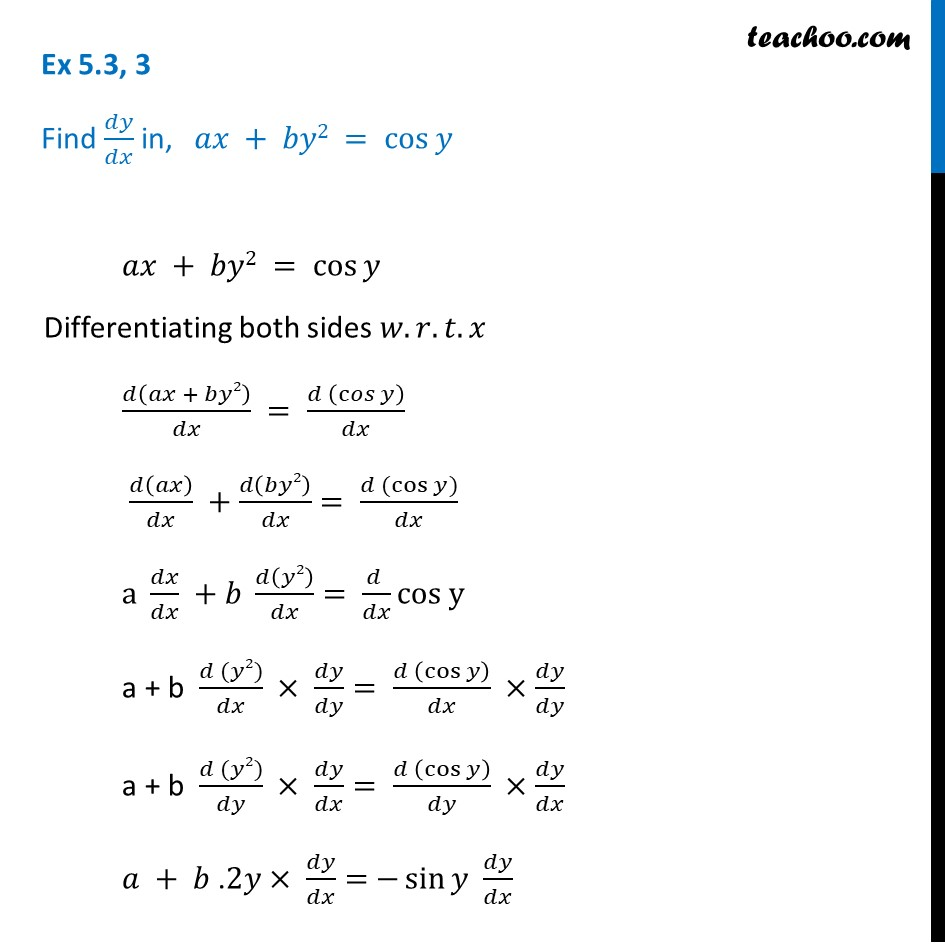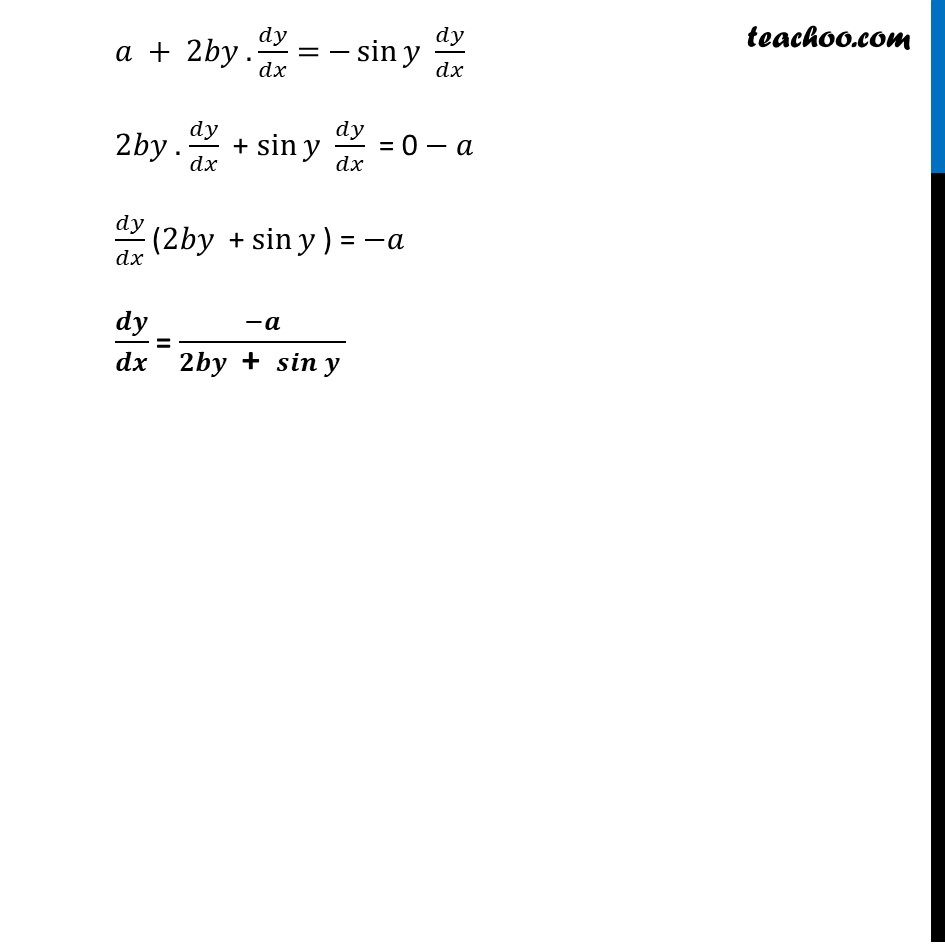Finding derivative of Implicit functions

Chapter 5 Class 12 Continuity and Differentiability
Concept wiseIntroducing your new favourite teacher - Teachoo Black, at only ₹83 per month

### Transcript

Ex 5.3, 3 Find 𝑑𝑦/𝑑𝑥 in, 𝑎𝑥 + 𝑏𝑦2 = cos⁡𝑦 𝑎𝑥 + 𝑏𝑦2 = cos⁡𝑦 Differentiating both sides 𝑤.𝑟.𝑡.𝑥 𝑑(𝑎𝑥 + 𝑏𝑦2)/𝑑𝑥 = (𝑑 (c𝑜𝑠⁡𝑦 ))/𝑑𝑥 𝑑(𝑎𝑥)/𝑑𝑥 +𝑑(𝑏𝑦2)/𝑑𝑥= (𝑑 〖(cos〗⁡𝑦))/𝑑𝑥 a 𝑑𝑥/𝑑𝑥 +𝑏 𝑑(𝑦2)/𝑑𝑥= (𝑑 )/𝑑𝑥 cos y a + b (𝑑 (𝑦2))/𝑑𝑥 × 𝑑𝑦/𝑑𝑦= (𝑑 (cos⁡𝑦 ))/𝑑𝑥 ×𝑑𝑦/𝑑𝑦 a + b (𝑑 (𝑦2))/𝑑𝑦 × 𝑑𝑦/𝑑𝑥= (𝑑 (cos⁡𝑦 ))/𝑑𝑦 ×𝑑𝑦/𝑑𝑥 𝑎 + 𝑏 .2𝑦× 𝑑𝑦/𝑑𝑥= −sin⁡〖𝑦 〗 𝑑𝑦/𝑑𝑥 𝑎 + 2𝑏𝑦 . 𝑑𝑦/𝑑𝑥= −sin⁡〖𝑦 〗 𝑑𝑦/𝑑𝑥 2𝑏𝑦 . 𝑑𝑦/𝑑𝑥 + sin⁡〖𝑦 〗 𝑑𝑦/𝑑𝑥 = 0 − 𝑎 𝑑𝑦/𝑑𝑥 (2𝑏𝑦 + sin⁡〖𝑦 〗) = −𝑎 𝒅𝒚/𝒅𝒙 = (−𝒂)/(𝟐𝒃𝒚 " + " 𝒔𝒊𝒏⁡〖𝒚 〗 )